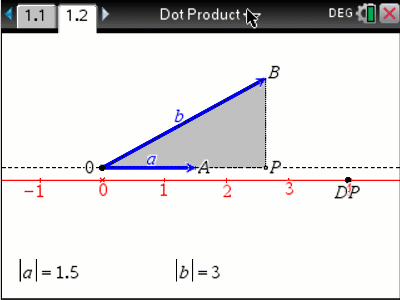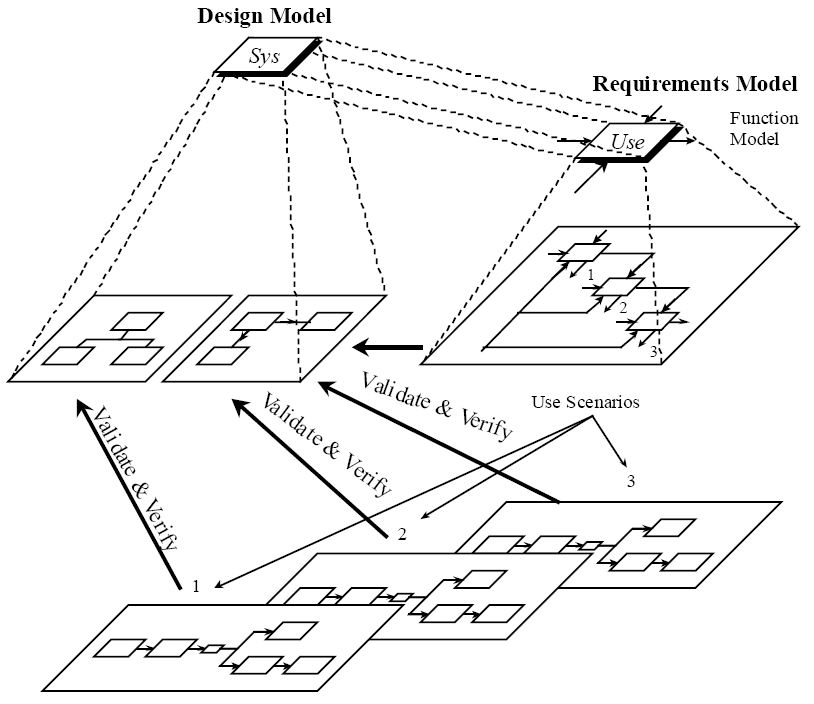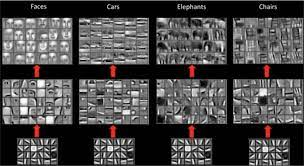Supervised learning is an example of observational inference – we’re just looking for associations between variables $$X$$ and $$Y$$. Aka, we’re just learning $$P(Y|X)$$.

I feel like this thread captures a really interesting divide / contrast of philosophies in machine learning research:

Researchers in speech recognition, computer vision, and natural language processing in the 2000s were obsessed with accurate representations of uncertainty.
1/N

— Yann LeCun (@ylecun) May 14, 2022

My goal now is to deeply understand the issues at hand in this thread. I found his mention of factor graphs in the shift to reasoning and planning AI was thought-provoking. I feel that causality and factor graphs and Bayesian and all that are very important. I just don’t know quite enough to put the pieces together yet.

### Machine Learning Interviews Book

This is a book that provides a comprehensive overview of machine learning interview process, including the various machine learning roles, interview pipeline, and practice interview questions.

Below are my (WIP) answers to some of the questions in the book. DISCLAIMER: While these answers are accurate to the best of my knowledge, I can’t guarantee their correctness.

### Machine Learning Interviews Book(Math > Algebra and (little) calculus > Vectors)

1. Dot product
1. [E] What’s the geometric interpretation of the dot product of two vectors?
The dot product $$\mathbf{x} \cdot \mathbf{y}$$ is the length of the projection of $$\mathbf{x}$$ onto the unit vector $$\mathbf{\hat{y}}$$; algebraically this is equal to $$\lvert\lvert \mathbf{x} \rvert\rvert \cos(\theta)$$, where $$\theta$$ is the angle between the vectors. Intuitively, the more the two vectors point in the same direction, the higher the dot product will be, as can be seen from the graphic below (where $$DP$$ shows the value of the dot product between $$a$$ and $$b$$.) When the vectors are orthogonal, the dot product is zero.2. [E] Given a vector $$u$$, find vector $$v$$ of unit length such that the dot product of $$u$$ and $$v$$ is maximum.
This would simply be the unit vector in the direction of $$u$$; namely, $$v = \frac{u}{\lvert\lvert u \rvert\rvert}$$.
2. Outer product
1. [E] Given two vectors $$a=[3,2,1]$$ and $$b=[−1,0,1]$$, calculate the outer product $$a^T b$$.
$$a^T b = \begin{bmatrix} 3 \\ 2 \\ 1 \end{bmatrix} \begin{bmatrix} 1 & 0 & 1 \end{bmatrix} = \begin{bmatrix} -3 & 0 & 3 \\ -2 & 0 & 2 \\ -1 & 0 & 1 \end{bmatrix}$$

We could also calculate this using numpy, like so:

import numpy as np
a = np.array([3,2,1])
b = np.array([-1,0,1])
print(np.outer(a, b))

[[-3  0  3]
[-2  0  2]
[-1  0  1]]

2. [M] Give an example of how the outer product can be useful in ML.
Potentially…in some sort of collaborative filtering system, you’d want an outer product between $$m$$ user vectors and $$n$$ item vectors, to produce a $$m \times n$$ similarity matrix?

Many applications seem to use the Kronecker product (generalization of the outer product to tensors) – don’t quite understand them yet, but here are a few:

There has to be something simpler that I’m missing.

3. [E] What does it mean for two vectors to be linearly independent?
A set of vectors is linearly independent if there is no way to nontrivially combine them into the zero vector. What this means is that there should e no way for the vectors to “cancel each other out,” so to speak; there are no “redundant” vectors.

For only two vectors, this occurs iff (1) neither of them is the zero vector (otherwise the other vector could easily be canceled out by a scalar multiple of $$0$$), and (2) they are not scalar multiples of each other (otherwise they could simply cancel each other out by multiplying one by a scalar such that it points in the opposite direction as the other.)

4. [M] Given two sets of vectors $$A=a_1,a_2,a_3,...,a_n$$
and $$B=b_1,b_2,b_3,...,b_m$$, How do you check that they share the same basis?

I think more precisely we’d want to determine whether the vector spaces spanned by $$A$$ and $$B$$ share the same basis. But for now, let’s just gloss over that minor distinction, and refer to the vector spaces as $$A$$ and $$B$$ as well. With that understanding, we can find a basis of $$A$$ by building a maximal linearly independent set, and then if that basis also spans $$B$$, then they share the same basis (in fact, that means that $$A$$ and $$B$$ span the same vector space.)
5. Given $$n$$ vectors, each of $$d$$ dimensions, what is the dimension of their span?
Not enough information. For example, let $$n = 2$$ and $$d = 2$$. Consider the vector space spanned by $$\{ \begin{bmatrix} 1 \\ 0 \end{bmatrix}, \begin{bmatrix} 2 \\ 0 \end{bmatrix}\}$$. This vector space is fully spanned by the basis $$\{ \begin{bmatrix} 1 \\ 0 \end{bmatrix}\}$$, making the dimension of the span $$1$$. However, if we consider the vector space spanned by $$\{ \begin{bmatrix} 2 \\ 3 \end{bmatrix}, \begin{bmatrix} 4 \\ 1 \end{bmatrix}\}$$, it is fully spanned by the basis $$\{ \begin{bmatrix} 1 \\ 0 \end{bmatrix}, \begin{bmatrix} 0 \\ 1 \end{bmatrix}\}$$, making the dimension of its span $$2$$. So, essentially it depends on the specific values of the vectors, and to what degree they’re linearly independent from each other (i.e. the size of the basis.)
6. Norms and metrics
1. [E] What’s a norm? What is $$L_0$$, $$L_1$$, $$L_2$$, $$L_{norm}$$?
Can be thought of as a vector’s distance from the origin. This becomes relevant in machine learning, when we want to objectively measure and minimize a scalar distance between the ground truth labels and model predictions.

So first, we get the vector distance between the ground truth and prediction, which we can call $$x$$. Then, we pass is through one of these norms to obtain a scalar distance:

$$L_0$$ “norm” is simply the total number of nonzero elements in a vector. If you consider $$0^0 = 0$$, then
$L_0(x) = \left|x_{1}\right|^{0}+\left|x_{2}\right|^{0}+\cdots+\left|x_{n}\right|^{0}$
$$L_1$$ norm: add up the absolute values of the elements.
$L_1(x) = \left|x_{1}\right|^{1}+\left|x_{2}\right|^{1}+\cdots+\left|x_{n}\right|^{1}$
$$L_2$$ norm: add up the squares of the elements.
$L_2(x) = \left|x_{1}\right|^{2}+\left|x_{2}\right|^{2}+\cdots+\left|x_{n}\right|^{2}$
…and so on.
$$L_\infty$$ norm is the equal to the maximum absolute value of a component of $$x$$. That is:
$L_\infty = \max_i |x_i|$

I can’t find anything on $$L_{norm}$$, so maybe that was a typo?

2. [M] How do norm and metric differ? Given a norm, make a metric. Given a metric, can we make a norm?
My understanding is that a norm is simply a mathematical operation on a vector, whereas a (loss) metric is measuring the distance between two things. Given a norm, let’s take the $$L_2$$ norm, we can easily make a metric, by plugging in a distance vector between ground truth and prediction, as shown in the previous question, and summing the squares of the components. A norm takes in a single variable (i.e. a vector,) whereas a metric takes in two (i.e. ground truth vector and predictions vector.)

### artificial intelligence

Just gonna link this to machine learning for now.

### compositionality

You have a set of reusable pieces that can be combined to form different, interesting things.

Related: 

Decomposition of programs into modular, reusable unit is one example of this.Some methodical activities, such as making breakfast, decompose well into modular activities as well:A machine learning example of compositionaity is that a computer vision model’s early layers will implement an edge detector, curve detector, etc., which are modular pieces that are then combined in various ways by higher-level layers to form dog detectors, car detectors, etc.One step towards compositionality is the idea of neuro-symbolic models. Combining logic-based systems with connectionist methods.

### ablation studies

Ablation studies are effectively using interventions (removing parts of your system) to reveal the underlying causal structure of your system. Francois Chollet (creator of Keras) writes about this being useful in a machine learning context: